﻿ 基于颗粒离散元法的连接键应变软化模型及其应用
«上一篇文章快速检索 高级检索

 力学学报2016, Vol. 48Issue (1): 76-85  DOI: 10.6052/0459-1879-15-2880

### 引用本文 [复制中英文]

[复制中文]
Feng Chun, Li Shihai, Liu Xiaoyu. PARTICLE-DEM BASED LINKED BAR STRAIN SOFTENING MODEL AND ITS APPLICATION[J]. Chinese Journal of Ship Research, 2016, 48(1): 76-85. DOI: 10.6052/0459-1879-15-288.
[复制英文]

### 文章历史

2015-07-30 收稿
2015-11-27 录用
2015-12-03 网络版发表

1 连接键模型 1.1 连接键的定义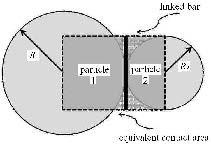图 1 连接键模型 Fig.1 Linked bar model

 ${A_{\rm{c}}} = \left\{ \begin{array}{l} \min (2{R_1},2{R_2})\;\;\;\;(2D)\\ \pi {[\min ({R_1},{R_2})]^2}\;\;(3D)\; \end{array} \right.$ (1)

 $\left. \begin{array}{l} {K_{\rm{n}}} = \bar E{A_{\rm{c}}}/({R_1} + {R_2})\\ {K_{\rm{s}}} = \bar G{A_{\rm{c}}}/({R_1} + {R_2}) \end{array} \right\}$ (2)

1.2 考虑应变软化效应的接触力及力矩计算

 $\left. {\begin{array}{*{20}{l}} {{F_{\rm{n}}}({t_1}) = {F_{\rm{n}}}({t_0}) - {K_{\rm{n}}}\Delta d{u_{\rm{n}}}}\\ {{F_{\rm{s}}}({t_1}) = {F_{\rm{s}}}({t_0}) - {K_{\rm{s}}}\Delta d{u_{\rm{s}}}} \end{array}} \right\}$ (3)

 $\left. \begin{array}{l} {M_{\rm{n}}}({t_1}) = {M_{\rm{n}}}({t_0}) - {K_{\rm{s}}}J\Delta d{\theta _{\rm{n}}}/{A_{\rm{c}}}\\ {M_{\rm{s}}}({t_1}) = {M_{\rm{s}}}({t_0}) - {K_{\rm{n}}}I\Delta d{\theta _{\rm{s}}}/{A_{\rm{c}}} \end{array} \right\}$ (4)

 $\left. \begin{array}{l} J = \pi {[\min (2{R_1},2{R_2})]^4}/32\\ I = J/2 \end{array} \right\}$ (5)

 $\left. \begin{array}{l} 如果\;\;\;\;\; - {F_{\rm{n}}}({t_1}) \ge T({t_0}){A_{\rm{c}}}\\ 那么\;\;\;\;{F_{\rm{n}}}({t_1}) = - T({t_0}){A_{\rm{c}}}\\ \;\;\;\;\;\;\;\;\;T({t_1}) = - {T_0}{\varepsilon _{\rm{p}}}/{\varepsilon _{\lim }} + {T_0} \end{array} \right\}$ (6)

 $\varepsilon _{\rm p} = \dfrac{\Delta u_{\rm n} }{R_1 + R_2 } - \dfrac{T(t_0 )}{\mathop{\bar {E}}\limits^{ \ }}$ (7)

 $\left. \begin{array}{l} 如果\;\;\;{F_{\rm{s}}}({t_1}) \ge {F_{\rm{n}}}({t_1})\tan \phi + c({t_0}){A_{\rm{c}}}\\ 那么\;\;\;{F_{\rm{s}}}({t_1}) = {F_{\rm{n}}}({t_1})\tan \phi + c({t_0}){A_{\rm{c}}}\\ \;\;\;\;\;\;\;c({t_1}) = - {c_0}{\gamma _{\rm{p}}}/{\gamma _{{\rm{lim}}}} + {c_0} \end{array} \right\}$ (8)

 $\gamma _{\rm p} = \dfrac{\Delta u_{\rm s} }{R_1 + R_2 } - \dfrac{c(t_0 ) + F_{\rm n} (t_1 )\tan (\phi) / A_{\rm c} }{\mathop{\bar{G}}\limits^{ \ } }$ (9)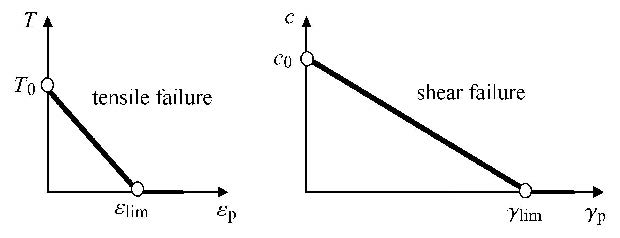图 2 抗拉强度及黏聚力的线性软化效应 Fig.2 Linear reduction effect of tensile strength and cohesion

 $\left. \begin{array}{l} \alpha = 1 - T(t)/{T_0}\\ \beta = 1 - c(t)/{c_0}\\ \chi = 1 - (1 - \alpha )(1 - \beta ) \end{array} \right\}$ (10)

 $\left. \begin{array}{l} [\frac{{ - {F_{\rm{n}}}}}{{{A_{\rm{c}}}}} + \frac{{|{M_{\rm{s}}}|}}{I}\min ({R_1},{R_2})] \ge {T_0}\;\;\;\;或\\ [\frac{{\left| {{F_{\rm{s}}}} \right|}}{{{A_{\rm{c}}}}} + \frac{{\left| {{M_{\rm{n}}}} \right|}}{J}\min ({R_1},{R_2})] \ge \frac{{{F_{\rm{n}}}}}{{{A_{\rm{c}}}}}\tan \phi + {c_0} \end{array} \right\}$ (11)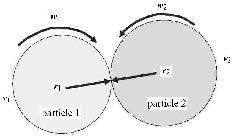图 3 颗粒的转动计算 Fig.3 Particle-rolling computation

 $\Delta {\pmb d} = ({\pmb w}_1 \times {\pmb r}_1 -{\pmb w}_2 \times {\pmb r}_2 )\Delta t + ({\pmb v}_1 - {\pmb v}_2 )\Delta t$ (12)
 ${\pmb M}_1 ={\pmb r}_1 \times {\pmb F}^{(G)} ,\ \ {\pmb M}_2 = - {\pmb r}_2 \times {\pmb F}^{(G)}$ (13)

1.3 单一连接键的计算精度验证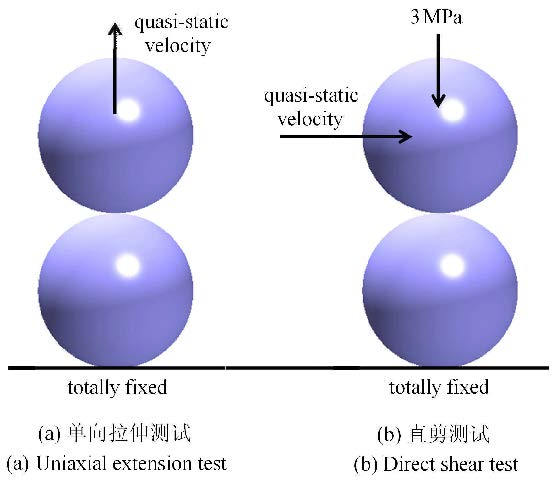图 4 单一连接键的精度测试 Fig.4 Precision test of single linked bar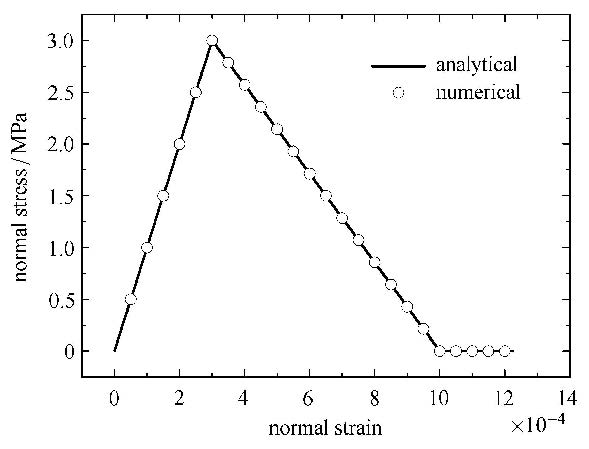图 5 拉应力与拉应变的关系 Fig.5 Relationship between normal stress and normal strain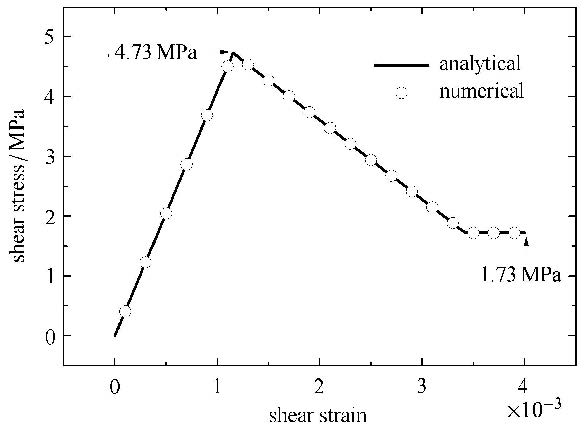图 6 剪应力与剪应变的关系 Fig.6 Relationship between shear stress and shear strai

 $\tau = c_0 + F_{\rm n} \tan (\phi) / A_{\rm c}$ (14)

1.4 平均配位数对宏观弹性特征的影响

 $\lambda = L_{\rm p} / L_{\rm e}$ (15)

 $\left. \begin{array}{l} {L_{\rm{p}}} = \sum\limits_{i = 1}^{{N_p}} {} (F_{\rm{n}}^i\Delta u_{\rm{n}}^i/2 + F_{\rm{s}}^i\Delta u_{\rm{s}}^i/2)\\ {L_{\rm{e}}} = {\rm{ }}\int {{\sigma _{ij}}{\varepsilon _{ij}}} /(2dV) \end{array} \right\}$ (16)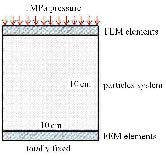图 7 颗粒系统模型 Fig.7 Model of particles system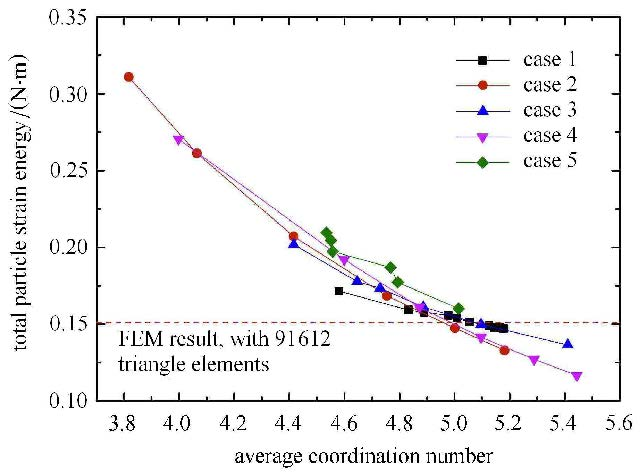图 8 颗粒应变能与平均配位数的关系 Fig.8 Relationship between particles' strain energy and average coordination number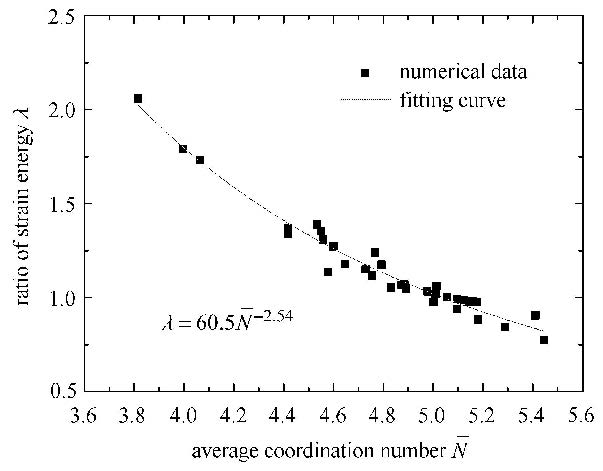图 9 细宏观应变能比值与平均配位数的拟合曲线 Fig.9 Fitting curve about ratio of micro and macro strain energy vs average coordination number

 $\lambda = 60.5\bar {N}^{ - 2.54}$ (17)

2 岩石单轴压缩过程数值分析 2.1 数值模型及计算工况

2.2 应力应变关系分析

6种工况下岩石试样的本构曲线如图 10所示. 由图可得，不同断裂应变下的岩石单轴压缩过程大致可以分为4个阶段，分别为线性上升段、非线性上升段、非线性下降段及缓变段. 6个工况下线性上升段的长度基本一致，该阶段的最大应力值约为5.9 MPa. 随着断裂应变的增大，非线性上升段呈现出逐渐增加的趋势；断裂应变为0时，非线性上升段仅为线性上升段的20%左右；而当断裂应变为2.4%时，非线性上升段的长度已达线性上升段的2倍以上. 随着断裂应变的增加，非线性下降段的下降速率有所增加，但在断裂应变为0.3%以后，下降速率的变化趋势并不明显. 6个工况下缓变段对应的应力值基本一致，约为0.4$\sim$2 MPa. 由图 10还可以看出，随着断裂应变的增加，本构曲线中的峰值强度及达到峰值强度时的应变也将逐渐增大.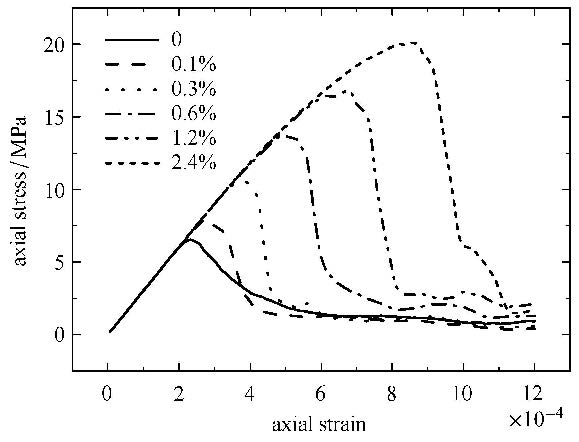图 10 不同断裂应变下的单轴压缩本构曲线 Fig.10 Constitutive curve of uniaxial compression test with different fracture strain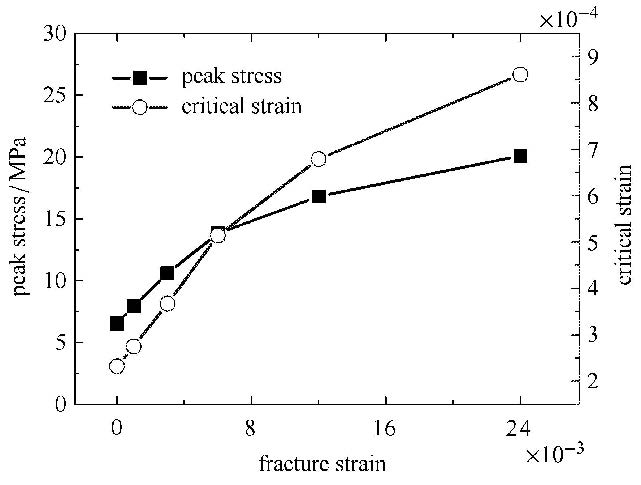图 11 峰值应力及临界应变随断裂应变的变化规律 Fig.11 Relationship between peak stress, critical strain and fracture strain
2.3 破坏模式及破裂程度分析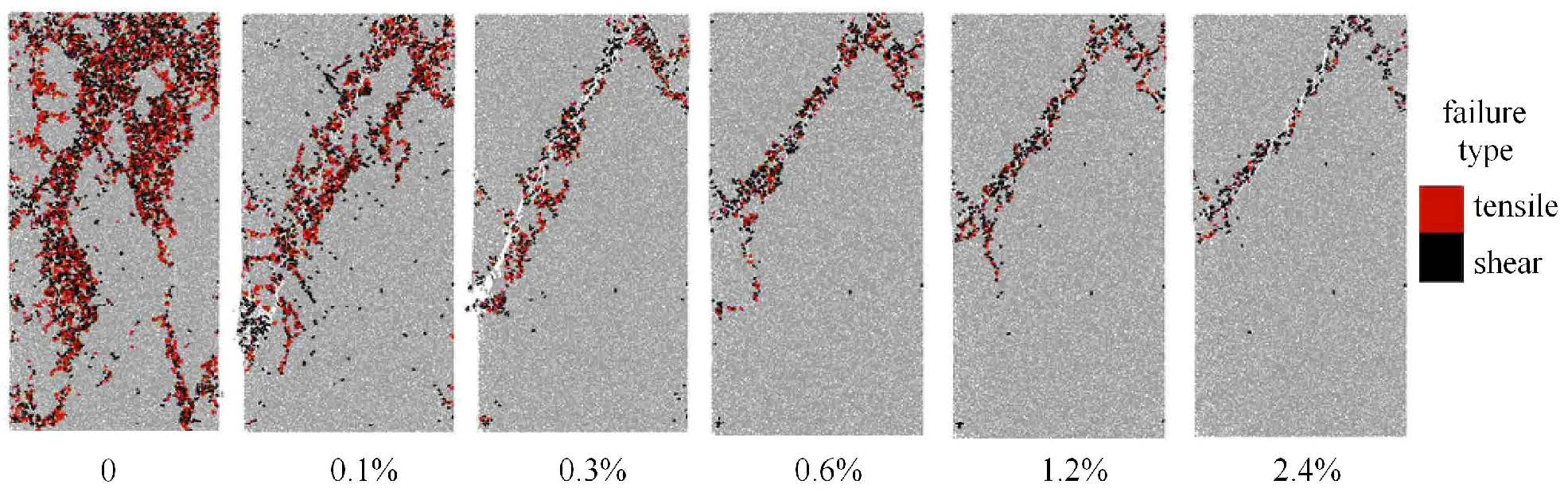图 12 不同断裂应变下的岩石试样破坏模式 Fig.12 Different failure mode of rock sample with different fracture strain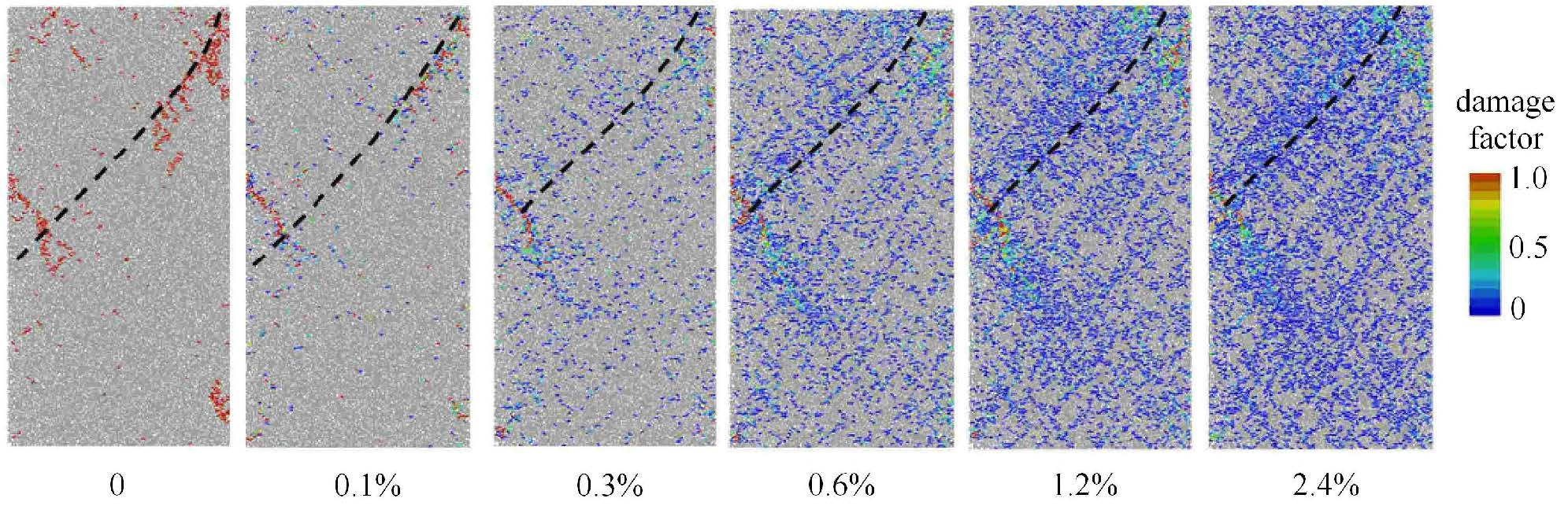图 13 不同断裂应变下岩石试样达到峰值强度时的联合损伤因子云图 Fig.13 Combined damage factor contour of rock sample under peak stress with different fracture strain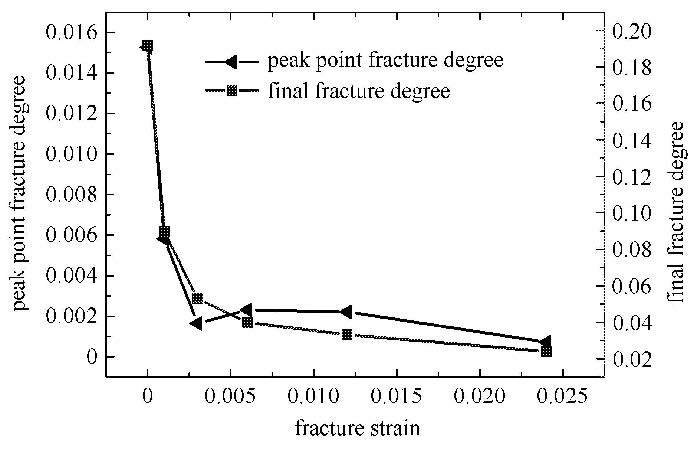图 14 峰值破裂度及终态破裂度与断裂应变的关系 Fig.14 Relationship between peak point fracture degree, final fracture degree and fracture strain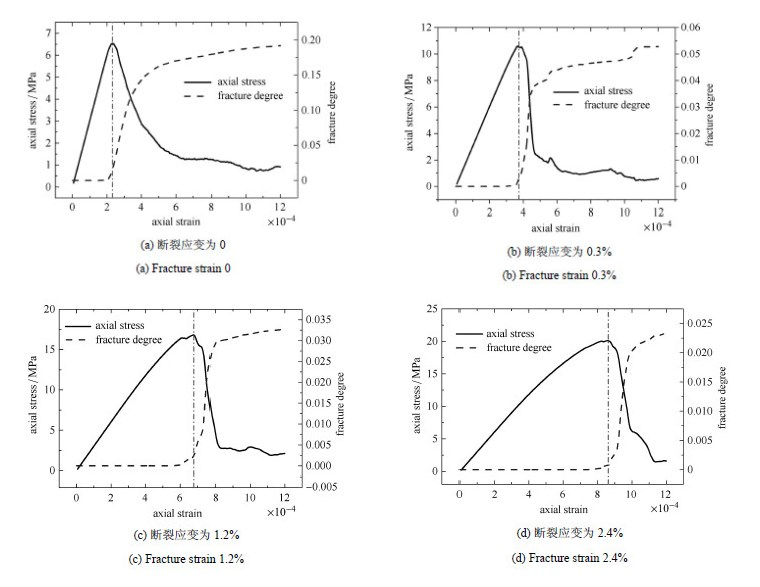图 15 典型断裂应变下轴向应力及破裂度随着轴向应变的变化规律 Fig.15 Relationship between axial stress, fracture degree and axial strain with typical fracture strain
3 结 论

(1) 不同断裂应变下的岩石单轴压缩过程大致可以分为4个阶段，分别为线性上升段、非线性上升段、非线性下降段及缓变段. 其中，线性上升段为弹性阶段，非线性上升段为均匀损伤阶段，非线性下降段为宏观主控裂缝的贯通阶段，缓变段为断裂后的试样沿着断口的滑移阶段.

(2) 随着断裂应变的增加，岩石的破裂模式逐渐由拉剪复合型破裂向单一压剪型破裂转化；且随着断裂应变的增加，峰值强度及达到峰值强度时的应变均逐渐增大，但峰值时的破裂度及终态时的破裂度将逐渐减小.

 1 徐泳, 孙其诚, 张凌等. 颗粒离散元法研究进展. 力学进展, 2003,33(2): 251-260 (Xu Yong, Sun Qicheng, Zhang Ling, et al. Advances in discrete element methods for particulate materials. Advances in Mechanics, 2003, 33(2): 251-260 (in Chinese)) 2 谭青, 徐孜军, 夏毅敏等. 盾构切刀作用下岩石动态响应机制的数 值模拟研究. 岩土工程学报, 2013, 35(2): 235-242 (Tan Qing, Xu Zijun, Xia Yimin, et al. Numerical simulation of dynamic response mechanism of rock by shield machine cutters. Chinese Journal of Geotechnical Engineering, 2013, 35(2): 235-242 (in Chinese)) 3 石崇, 王盛年, 刘琳. 地震作用下陡岩崩塌颗粒离散元数值模拟 研究. 岩石力学与工程学报, 2013, 32(S1): 2798-2805 (Shi Chong, Wang Shengnian, Liu Lin. Research of avalanche disaster numerical simulation based on granular discrete element method of high-steep slope under seismic loads. Chinese Journal of Rock Mechanics and Engineering, 2013, 32(S1): 2798-2805 (in Chinese)) 4 张社荣, 孙博, 王超等. 双轴压缩试验下岩石裂纹扩展的离散 元分析. 岩石力学与工程学报, 2013, 32(S2): 3083-3091 (Zhang Sherong, Sun Bo, Wang Chao, et al. Discrete element analysis of crack propagation in rocks under biaxial compression. Chinese Journal of Rock Mechanics and Engineering, 2013, 32(S2): 3083-3091 (in Chinese)) 5 Ji SY, Di SC. Discrete element modeling of acoustic emission in rock fracture. Theoretical and Applied Mechanics Letters, 2013,3(2): 021009-021009-5 6 Utili S, Nova R. DEM analysis of bonded granular geomaterials. International Journal for Numerical and Analytical Methods in Geomechanics,2008, 32(17): 1997-2031 7 Oñate E, Zarate F, Miquel J, et al. A local constitutive model for the discrete element method. Application to geomaterials and concrete. Computational Particle Mechanics, 2015, 2: 139-160 8 Huang H, Lecampion B, Detournay E. Discrete element modeling of tool—rock interaction I: Rock cutting. International Journal for Numerical and Analytical Methods in Geomechanics, 2013, 37(13):1913-1929 9 Huang H, Detournay E. Discrete element modeling of tool—rock interaction II: rock indentation. International Journal for Numerical and Analytical Methods in Geomechanics, 2013, 37(13): 1930-1947 10 Yang BD, Jiao Y, Lei S. A study on the e ects of microparameters on macroproperties for specimens created by bonded particles. Engineering Computations, 2006, 23(6): 607-631 11 Yoon J. Application of experimental design and optimization to PFC model calibration in uniaxial compression simulation. International Journal of Rock Mechanics and Mining Sciences, 2007, 44(6): 871-889 12 Coetzee CJ, Els DNJ. Calibration of discrete element parameters and the modelling of silo discharge and bucket filling. Computers and Electronics in Agriculture, 2009, 65(2): 198-212 13 Wang Y, Tonon F. Calibration of a discrete element model for intact rock up to its peak strength. International Journal for Numerical and Analytical Methods in Geomechanics, 2010, 34(5): 447-469 14 Itasca Consulting Group Inc. PFC3D (Particle Flow Code in 3 Dimensions), Version 4.0, User's Manual. USA: Itasca Consulting Group Inc, 2003 15 Jing LR, Stephansson O. Fundamentals of Discrete Element Methods for Rock Engineering: Theory and Applications. Elsevier, Amsterdam,2007 16 吴顺川, 张晓平, 刘洋. 基于颗粒元模拟的含软弱夹层类土质边 坡变形破坏过程分析. 岩土力学, 2008, 29(11): 2899-2904 (Wu Shunchuan, Zhang Xiaoping, Liu Yang. Analysis of failure process of similar soil slope with weak intercalated layer based on particle flow simulation. Rock and Soil Mechanics, 2008, 29(11): 2899-2904 (in Chinese)) 17 赵国彦, 戴兵, 马驰. 平行黏结模型中细观参数对宏观特性影 响研究. 岩石力学与工程学报, 2012, 31(7): 1491-1498 (Zhao Guoyan,Dai Bing,Ma Chi. Study of e ects of microparameters on macroproperties for parallel bonded model. Chinese Journal of Rock Mechanics and Engineering, 2012, 31(7): 1491-1498 (in Chinese)) 18 祁原, 黄俊杰, 陈明祥. 可破碎颗粒体在动力载荷下的耗能特性. 力学学报, 2015, 47(2): 252-259 (Qi Yuan, Huang Junjie, Chen Mingxiang. Energy dissipation characteristics of crushable granules under dynamic excitations. Chinese Journal of Theoretical and Applied Mechanics, 2015, 47(2): 252-259 (in Chinese)) 19 Gri ths DV, Mustoe GGW. Modelling of elastic continua using a grillage of structural elements based on discrete element concepts . International Journal for Numerical Methods in Engineering, 2001,50(7): 1759-1775 20 Chang CS, Shi Q, Liao CL. Elastic constants for granular materials modeled as first-order strain-gradient continua. International Journal of Solids and Structures, 2003, 40(21): 5565-5582 21 Kruyt NP, Rothenburg L. Micromechanical bounds for the e ective elastic moduli of granular materials. International Journal of Solids and Structures, 2002, 39(2): 311-324
PARTICLE-DEM BASED LINKED BAR STRAIN SOFTENING MODEL AND ITS APPLICATION
Feng Chun, Li Shihai, Liu Xiaoyu
Key Laboratory for Mechanics in Fluid Solid Coupling Systems, Institute of Mechanics, Chinese Academy of Sciences, Beijing 100190, China
Abstract: Based on finite contact assumption between particles, a linked bar model to transmit the force and moment between particles is proposed. To represent the plastic, damage and fracture process of linked bar, the Mohr-Coulomb model and maximal tensile stress model considering strain softening e ect is introduced. The numerical results of uniaxial extension test and direct shear test with single linked bar show the accuracy of the model. The relationship between equivalent macro strain energy of particles system and the average coordination number is studied. Numerical results show that, for 2D particles system, when the average coordination number equals 5, the equivalent macro strain energy of particles system coincides well with the strain energy computed by approaches based on continuous media (i.e. FEM). The uniaxial compression process of rock is simulated based on the linked bar strain softening model. The results show that, the strain-stress curve of rock during uniaxial compression could be divided to four stages, which are linear stage, hardening stage, softening stage and sliding stage, and the relationships between four stages and damage fracture status of rock are also studied. From the results, with the increase of fracture strain, the failure mode of rock changes from tensile-shear composite fracture pattern to purely compression shear fracture pattern. With the increase of fracture strain, the peak stress and corresponding critical strain increases gradually, however, the fracture degree at peak point and at final state decreases gradually.
Key words: particle flow    linked bar    strain softening    uniaxial compression    damage fracture